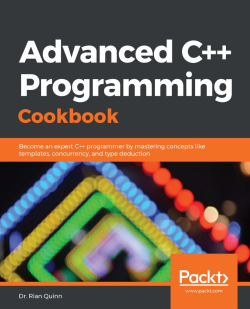3 (1 reviews total)
By Dr. Rian Quinn
• \$28.99 eBook
• \$41.99 Print + eBook
What do you get with a Packt Subscription?

• Constantly updated with 100+ new titles each month
• Breadth and depth in over 1,000+ technologies

If you think you've mastered C++ and know everything it takes to write robust applications, you'll be in for a surprise. With this book, you'll gain comprehensive insights into C++, covering exclusive tips and interesting techniques to enhance your app development process.

You'll kick off with the basic principles of library design and development, which will help you understand how to write reusable and maintainable code. You'll then discover the importance of exception safety, and how you can avoid unexpected errors or bugs in your code. The book will take you through the modern elements of C++, such as move semantics, type deductions, and coroutines. As you advance, you'll delve into template programming - the standard tool for most library developers looking to achieve high code reusability. You'll explore the STL and learn how to avoid common pitfalls while implementing templates. Later, you'll learn about the problems of multithreaded programming such as data races, deadlocks, and thread starvation. You'll also learn high-performance programming by using benchmarking tools and libraries. Finally, you'll discover advanced techniques for debugging and testing to ensure code reliability.

By the end of this book, you'll have become an expert at C++ programming and will have gained the skills to solve complex development problems with ease.

Publication date:
January 2020
Publisher
Packt
Pages
454
ISBN
9781838559915

# Using Exceptions for Error Handling

In this chapter, we will learn some advanced C++ exception handling techniques. We assume here that you have a basic understanding of how to throw as well as catch a C++ exception. Instead of focusing on the basics of C++ exceptions, this chapter will teach you some of the more advanced techniques of C++ exception handling. This includes the proper use of the noexcept specifier and the noexcept operator so that you can properly mark your APIs as either possibly throwing an exception or explicitly not throwing a C++ exception, instead of calling std::terminate() when an error occurs that cannot be handled.

This chapter will also explain what the term Resource Acquisition is Initialization (RAII) is and how it complements C++ exception handling. We will also discuss why you should never throw a C++ exception from a class's destructor and how to handle these types of issues. Finally, we will look at how to create your own custom C++ exceptions including providing some basic guidelines on what to do and what not to do when creating your own exceptions.

From the information provided in this chapter, you will gain a better understanding of how C++ exceptions work under the hood and the types of things that can be done with C++ exceptions to build more robust and reliable C++ programs.

The recipes in this chapter are as follows:

• Using the noexcept specifier
• Using the noexcept operator
• Using RAII
• Learning why to never throw exceptions in destructors
• Easily creating your own exception classes

# Technical requirements

To compile and run the examples in this chapter, you must have administrative access to a computer running Ubuntu 18.04 with a functional internet connection. Before running these examples, you must install the following:

`sudo apt-get install build-essential git cmake`

If this is installed on any operating system other than Ubuntu 18.04, then GCC 7.4 or higher and CMake 3.6 or higher will be required.

# Using the noexcept specifier

The noexcept specifier is used to tell the compiler whether a function may or may not throw a C++ exception. If a function is marked with the noexcept specifier, it is not allowed to throw an exception and, if it does, std::terminate() will be called when the exception is thrown. If the function doesn't have the noexcept specifier, exceptions can be thrown as normal.

In this recipe, we will explore how to use the noexcept specifier in your own code. This specifier is important because it is a contract between the API that you are creating and the user of the API. When the noexcept specifier is used, it tells the user of the API that they do not need to consider exceptions when using the API. It also tells the author that if they add the noexcept specifier to their API, they have to ensure that no exceptions are thrown, which, in some cases, requires the author to catch all possible exceptions and either handle them or call std::terminate() if the exception cannot be handled. Also, there are certain operations, such as std::move, where exceptions cannot be thrown without the fear of corruption as a move operation oftentimes cannot be safely reversed if an exception is thrown. Finally, with some compilers, adding noexcept to your APIs will reduce the overall size of the function, resulting in a smaller overall application.

Before beginning, please ensure that all of the technical requirements are met, including installing Ubuntu 18.04 or higher and running the following in a Terminal window:

`> sudo apt-get install build-essential git cmake`

This will ensure your operating system has the proper tools to compile and execute the examples in this recipe. Once this is complete, open a new Terminal. We will use this Terminal to download, compile, and run our examples.

# How to do it...

To try this recipe, perform the following steps:

1. From a new Terminal, run the following to download the source code:
`> cd ~/> git clone https://github.com/PacktPublishing/Advanced-CPP-CookBook.git> cd Advanced-CPP-CookBook/chapter02`
1. To compile the source code, run the following:
`> mkdir build && cd build> cmake ..> make recipe01_examples`
1. Once the source code is compiled, you can execute each example in this recipe by running the following commands:
`> ./recipe01_example01The answer is: 42> ./recipe01_example02terminate called after throwing an instance of 'std::runtime_error'what(): The answer is: 42Aborted> ./recipe01_example03The answer is: 42> ./recipe01_example04terminate called after throwing an instance of 'std::runtime_error'what(): The answer is: 42Aborted> ./recipe01_example05foo: 18446744069414584320foo: T is too large`

In the next section, we will step through each of these examples and explain what each example program does and how it relates to the lessons being taught in this recipe.

# How it works...

First, let's briefly review how C++ exceptions are thrown and caught. In the following example, we will throw an exception from a function and then catch the exception in our main() function:

`#include <iostream>#include <stdexcept>void foo(){    throw std::runtime_error("The answer is: 42");}int main(void){    try {        foo();    }    catch(const std::exception &e) {        std::cout << e.what() << '\n';    }    return 0;}`

As shown in the preceding example, we created a function called foo() that throws an exception. This function is called in our main() function inside a try/catch block, which is used to catch any exceptions that might be thrown by the code executed inside the try block, which in this case is the foo() function. When the exception is thrown by the foo() function, it is successfully caught and outputted to stdout.

All of this works because we did not add the noexcept specifier to the foo() function. By default, a function is allowed to throw an exception, just as we did in this example. In some cases, however, we do not want to allow exceptions to be thrown, depending on how we expect a function to execute. Specifically, how a function handles exceptions can be defined as the following (known as exception safety):

• No-throw guarantee: The function cannot throw an exception, and if an exception is thrown internally, the exception must be caught and handled, including allocation failures.
• Strong exception safety: The function can throw an exception, and if an exception is thrown, any state that was modified by the function is rolled back or undone with no side effects.
• Basic exception safety: The function can throw an exception, and if an exception is thrown, any state that was modified by the function is rolled back or undone, but side effects are possible. It should be noted that these side effects do not include invariants, meaning the program is in a valid, non-corrupted state.
• No exception safety: The function can throw an exception, and if an exception is thrown, the program could enter a corrupted state.

In general, if a function has a no-throw guarantee, it is labeled with noexcept; otherwise, it is not. An example of why exception safety is so important is with std::move. For example, suppose we have two instances of std::vector and we wish to move one vector into another. To perform the move, std::vector might move each element of the vector from one instance to the other. If the object is allowed to throw when it is moved, the vector could end up with an exception in the middle of the move (that is, half of the objects in the vector are moved successfully). When the exception occurs, std::vector would obviously attempt to undo the moves that it has already performed by moving these back to the original vector before returning the exception. The problem is, attempting to move the objects back would require std::move(), which could throw and exception again, resulting in a nested exception. In practice, moving one std::vector instance to another doesn't actually perform an object-by-object move, but resizing does, and, in this specific issue, the standard library requires the use of std::move_if_noexcept to handle this situation to provide exception safety, which falls back to a copy when the move constructor of an object is allowed to throw.

The noexcept specifier is used to overcome these types of issues by explicitly stating that the function is not allowed to throw an exception. This not only tells the user of the API that they can safely use the function without fear of an exception being thrown and potentially corrupting the execution of the program, but it also forces the author of the function to safely handle all possible exceptions or call std::terminate(). Although noexcept, depending on the compiler, also provides optimizations by reducing the overall size of the application when defined, its main use is to state the exception safety of a function such that other functions can reason about how a function will execute.

In the following example, we add the noexcept specifier to our foo() function defined earlier:

`#include <iostream>#include <stdexcept>void foo() noexcept{    throw std::runtime_error("The answer is: 42");}int main(void){    try {        foo();    }    catch(const std::exception &e) {        std::cout << e.what() << '\n';    }    return 0;}`

When this example is compiled and executed, we get the following: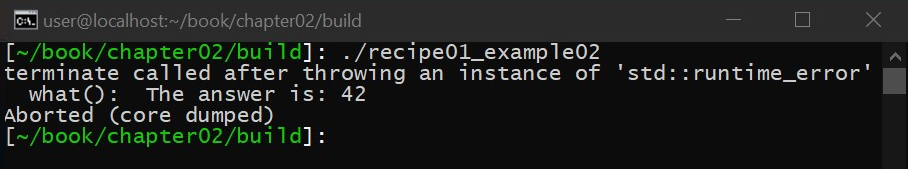As shown in the preceding example, the noexcept specifier was added, which tells the compiler that foo() is not allowed to throw an exception. Since, however, the foo() function does throw an exception, when it is executed, std::terminate() is called. In fact, in this example, std::terminate() will always be called, which is something the compiler is able to detect and warn about.

Calling std::terminate() is obviously not the desired outcome of a program. In this specific case, since the author has labeled the function as noexcept, it is up to the author to handle all possible exceptions. This can be done as follows:

`#include <iostream>#include <stdexcept>void foo() noexcept{    try {        throw std::runtime_error("The answer is: 42");    }    catch(const std::exception &e) {        std::cout << e.what() << '\n';    }}int main(void){    foo();    return 0;}`

As shown in the preceding example, the exception is wrapped in a try/catch block to ensure the exception is safely handled before the foo() function completes its execution. Also, in this example, only exceptions that originate from std::exception() are caught. This is the author's way of saying which types of exceptions can be safely handled. If, for example, an integer was thrown instead of std::exception(), std::terminate() would still be executed automatically since noexcept was added to the foo() function. In other words, as the author, you are only required to handle the exceptions that you can, in fact, safely handle. The rest will be sent to std::terminate() for you; just understand that, by doing this, you change the exception safety of the function. If you intend for a function to be defined with a no-throw guarantee, the function cannot throw an exception at all.

It should also be noted that if you mark a function as noexcept, you need to not only pay attention to exceptions that you throw but also to the functions that may throw themselves. In this case, std::cout is being used inside the foo() function, which means the author has to either knowingly ignore any exceptions that std::cout could throw, which would result in a call to std::terminate() (which is what we are doing here), or the author needs to identify which exceptions std::cout could throw and attempt to safely handle them, including exceptions such as std::bad_alloc.

The std::vector.at() function throws an std::out_of_range() exception if the provided index is out of bounds with respect to the vector. In this case, the author can catch this type of exception and return a default value, allowing the author to safely mark the function as noexcept.

The noexcept specifier is also capable of acting as a function, taking a Boolean expression, as in the following example:

`#include <iostream>#include <stdexcept>void foo() noexcept(true){    throw std::runtime_error("The answer is: 42");}int main(void){    try {        foo();    }    catch(const std::exception &e) {        std::cout << e.what() << '\n';    }    return 0;}`

This results in the following when executed: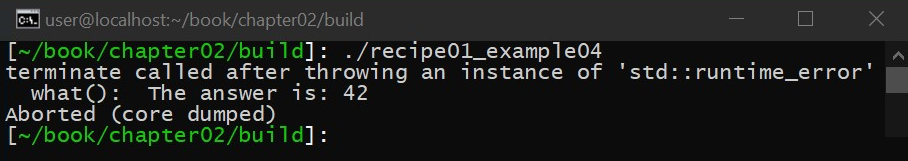As shown in the preceding example, the noexcept specifier was written as noexcept(true). If the expression evaluates to true, it is as if noexcept was provided. If the expression evaluates to false, it is as if the noexcept specifier was left out, allowing exceptions to be thrown. In the preceding example, the expression evaluates to true, which means that the function is not allowed to throw an exception, which results in std::terminate() being called when foo() throws an exception.

Let's look at a more complicated example to demonstrate how this can be used. In the following example, we will create a function called foo() that will shift an integer value by 32 bits and cast the result to a 64-bit integer. This example will be written using template metaprogramming, allowing us to use this function on any integer type:

`#include <limits>#include <iostream>#include <stdexcept>template<typename T>uint64_t foo(T val) noexcept(sizeof(T) <= 4){    if constexpr(sizeof(T) <= 4) {        return static_cast<uint64_t>(val) << 32;    }    throw std::runtime_error("T is too large");}int main(void){    try {        uint32_t val1 = std::numeric_limits<uint32_t>::max();        std::cout << "foo: " << foo(val1) << '\n';        uint64_t val2 = std::numeric_limits<uint64_t>::max();        std::cout << "foo: " << foo(val2) << '\n';    }    catch(const std::exception &e) {        std::cout << e.what() << '\n';    }    return 0;}`

This results in the following when executed: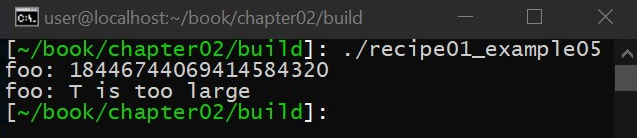As shown in the preceding example, the issue with the foo() function is that if the user provides a 64-bit integer, it cannot shift by 32 bits without generating an overflow. If the integer provided, however, is 32 bits or less, the foo() function is perfectly safe. To implement the foo() function, we used the noexcept specifier to state that the function is not allowed to throw an exception if the provided integer is 32 bits or less. If the provided integer is greater than 32 bits, an exception is allowed to throw, which, in this case, is an std::runtime_error() exception stating that the integer is too large to be safely shifted.

# Using the noexcept operator

The noexcept operator is a compile-time check that is used to ask the compiler whether a function is labeled noexcept or not. With C++17, this can be paired with a compile-time if statement (that is, an if statement that is evaluated at compile time and that can be used to add/remove code from an executable during compilation) to change the semantics of a program based on whether or not a function is allowed to throw an exception.

In this recipe, we will explore how to use the noexcept operator in your own code. This operator is important because, in some cases, you may not know whether a function is capable of throwing an exception by simply looking at its definition. For example, if a function uses the noexcept specifier, your code might not be able to determine whether the function will throw, as you might not know—based on the function's inputs—what the noexcept specifier will evaluate to. The noexcept operator provides you with a mechanism to handle these types of scenarios, which is essential, especially when metaprogramming.

Before beginning, please ensure that all of the technical requirements are met, including installing Ubuntu 18.04 or higher and running the following in a Terminal window:

`> sudo apt-get install build-essential git cmake`

This will ensure your operating system has the proper tools to compile and execute the examples in this recipe. Once this is complete, open a new Terminal. We will use this Terminal to download, compile, and run our examples.

# How to do it...

Perform the following steps to try the recipe:

1. From a new Terminal, run the following to download the source code:
`> cd ~/> git clone https://github.com/PacktPublishing/Advanced-CPP-CookBook.git> cd Advanced-CPP-CookBook/chapter02`
1. To compile the source code, run the following:
`> mkdir build && cd build> cmake ..> make recipe02_examples`

1. Once the source code is compiled, you can execute each example in this recipe by running the following commands:
`> ./recipe02_example01could foo throw: true> ./recipe02_example02could foo throw: truecould foo throw: truecould foo throw: falsecould foo throw: false> ./recipe02_example03terminate called after throwing an instance of 'std::runtime_error'what(): The answer is: 42Aborted> ./recipe02_example04> ./recipe02_example05terminate called after throwing an instance of 'std::runtime_error'what(): The answer is: 42Aborted> ./recipe02_example06could foo throw: truecould foo throw: true`

In the next section, we will step through each of these examples and explain what each example program does and how it relates to the lessons being taught in this recipe.

# How it works...

The noexcept operator is used to determine whether a function can throw. Let's start with a simple example:

`#include <iostream>#include <stdexcept>void foo(){    std::cout << "The answer is: 42\n";}int main(void){    std::cout << std::boolalpha;    std::cout << "could foo throw: " << !noexcept(foo()) << '\n';    return 0;}`

This results in the following: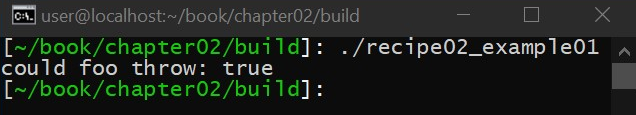As shown in the preceding example, we defined a foo() function that outputs to stdout. We don't actually execute foo() but, instead, we use the noexcept operator to check to see whether the foo() function could throw. As you can see, the answer is yes; this function can throw. This is because we did not label the foo() function with noexcept, and, as stated in the previous recipe, functions can throw by default.

It should also be noted that we added ! to the noexcept expression. This is because noexcept returns true if the function is labeled noexcept, which means that the function is not allowed to throw. However, in our example, we are not asking whether the function cannot throw, but instead we are asking whether the function can throw, hence the logical Boolean reversal.

Let's expand upon this by adding a couple more functions to our example. Specifically, in the following example, we will add some functions that throw as well as some functions that are labeled noexcept:

`#include <iostream>#include <stdexcept>void foo1(){    std::cout << "The answer is: 42\n";}void foo2(){    throw std::runtime_error("The answer is: 42");}void foo3() noexcept{    std::cout << "The answer is: 42\n";}void foo4() noexcept{    throw std::runtime_error("The answer is: 42");}int main(void){    std::cout << std::boolalpha;    std::cout << "could foo throw: " << !noexcept(foo1()) << '\n';    std::cout << "could foo throw: " << !noexcept(foo2()) << '\n';    std::cout << "could foo throw: " << !noexcept(foo3()) << '\n';    std::cout << "could foo throw: " << !noexcept(foo4()) << '\n';    return 0;}`

This results in the following: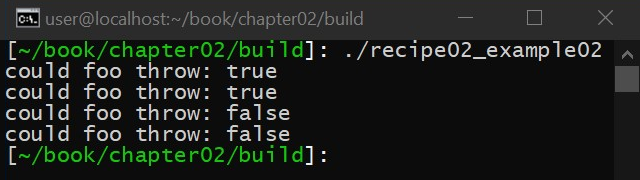As shown in the preceding example, if a function is labeled with noexcept, the noexcept operator returns true (which, in our example, outputs false). More importantly, a keen observer would notice that the functions that throw exceptions do not change the output of the noexcept operator. That is, the noexcept operator returns false if a function can throw an exception, not if it will throw an exception. This is important as the only way to know whether a function will throw an exception is to execute it. The only thing the noexcept specifier states is whether or not an exception is allowed to be thrown by the function. It doesn't state whether or not an exception will be thrown. By extension, the noexcept operator doesn't tell you whether the function will throw or not but instead tells you whether the function is labeled with the noexcept specifier (and, more importantly, what the noexcept specifier evaluates to).

Before we attempt to use the noexcept specifier in a more realistic example, let's look at the following example:

`#include <iostream>#include <stdexcept>void foo(){    throw std::runtime_error("The answer is: 42");}int main(void){    foo();}`

As shown in the preceding example, we have defined a foo() function that throws, and then we call this function from our main function, resulting in std::terminate() being called because we didn't handle the exception before leaving the program. In a more complicated setting, we might not know whether foo() throws or not, and, as a result, we may not want to add the additional overhead of exception handling if it is not needed. To better explain this, let's examine the resulting assembly code for the main() function for this example: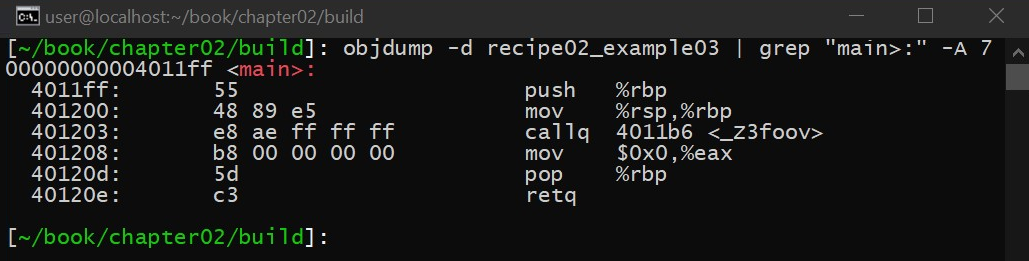As you can see, the main function is simple and doesn't contain any additional logic outside of calling the foo function. Specifically, the main function doesn't have any catch logic in it.

Now, let's use the noexcept operator in a more concrete example:

`#include <iostream>#include <stdexcept>void foo(){    throw std::runtime_error("The answer is: 42");}int main(void){    if constexpr(noexcept(foo())) {        foo();    }    else {        try {            foo();        }        catch (...)        { }    }}`

As shown in the preceding example, we use the noexcept operator in conjunction with the constepxr operator in the if statement that was added in C++17. This allows us to ask the compiler whether foo() is allowed to throw. If it is, we execute the foo() function inside a try/catch block so that we can handle any possible exceptions as needed. If we examine the assembly of this function, as shown in the following screenshot, we can see that some additional catch logic was added to the resulting binary to handle the exceptions as needed: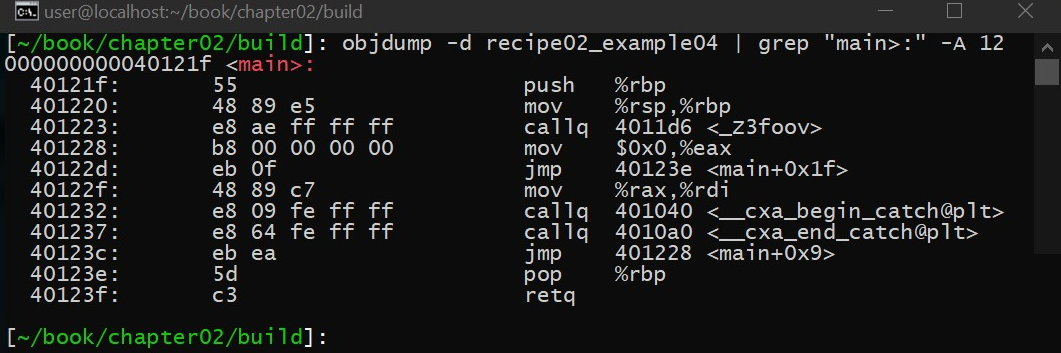Now, let's take this same example one step further by stating that the foo() function is not allowed to throw using the noexcept specifier:

`#include <iostream>#include <stdexcept>void foo() noexcept{    throw std::runtime_error("The answer is: 42");}int main(void){    if constexpr(noexcept(foo())) {        foo();    }    else {        try {            foo();        }        catch (...)        { }    }}`

As shown in the preceding example, the program calls std::terminate() since the foo() function was labeled noexcept. Furthermore, if we look at the resulting assembly, we can see that the main() function no longer contains the additional try/catch logic, which means that our optimization worked: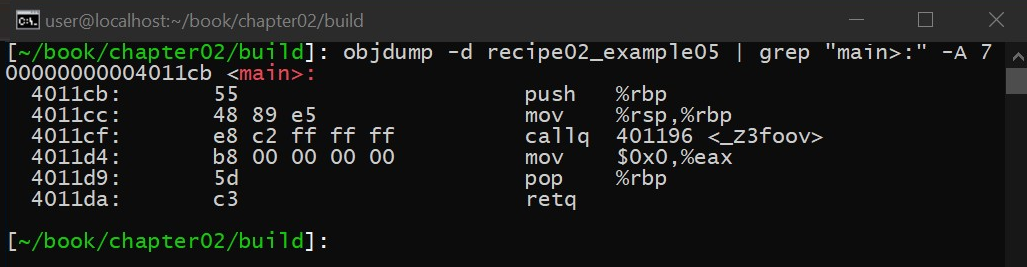Finally, we might possibly not know how to label our own function if we do not know whether a function that was called can throw or not. Let's look at the following example to demonstrate this issue:

`#include <iostream>#include <stdexcept>void foo1(){    std::cout << "The answer is: 42\n";}void foo2() noexcept(noexcept(foo1())){    foo1();}int main(void){    std::cout << std::boolalpha;    std::cout << "could foo throw: " << !noexcept(foo1()) << '\n';    std::cout << "could foo throw: " << !noexcept(foo2()) << '\n';}`

This results in the following: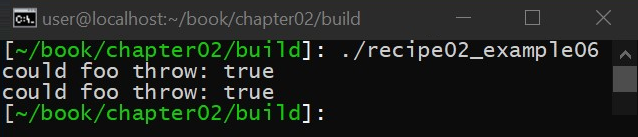As shown in the preceding example, the foo1() function is not labeled with the noexcept specifier, which means it is allowed to throw an exception. In foo2(), we want to ensure that our noexcept specifier is correct but we call foo1(), and, in this example, we assume that we don't know whether foo1() is noexcept or not.

To ensure foo2() is labeled properly, we combine the lessons learned in this recipe and the previous one to mark the function properly. Specifically, we use the noexcept operator to tell us whether the foo1() function will throw, and then we use the noexcept specifier's Boolean expression syntax to use the results of the noexcept operator to label foo2() as noexcept or not. If foo1() is labeled with noexcept, the noexcept operator will return true, resulting in foo2() being marked as noexcept(true), which is the same as simply stating noexcept. If foo1() is not labeled as noexcept, the noexcept operator will return false, in which case the noexcept specifier will be labeled as noexcept(false), which is the same as not adding the noexcept specifier (that is, the function is allowed to throw an exception).

# Using RAII

RAII is a programming principle that states that a resource is tied to the lifetime of the object that acquired the resource. RAII is a powerful feature of the C++ language that really helps to set C++ apart from C, helping to prevent resource leaks and general instability.

In this recipe, we will dive into how RAII works and how RAII can be used to ensure that C++ exceptions do not introduce resource leaks. RAII is a critical technology for any C++ application and should be used whenever possible.

Before beginning, please ensure that all of the technical requirements are met, including installing Ubuntu 18.04 or higher and running the following in a Terminal window:

`> sudo apt-get install build-essential git cmake`

This will ensure your operating system has the proper tools to compile and execute the examples in this recipe. Once this is complete, open a new Terminal. We will use this Terminal to download, compile, and run our examples.

# How to do it...

You need to perform the following steps to try the recipe:

1. From a new Terminal, run the following to download the source code:
`> cd ~/> git clone https://github.com/PacktPublishing/Advanced-CPP-CookBook.git> cd Advanced-CPP-CookBook/chapter02`
1. To compile the source code, run the following:
`> mkdir build && cd build> cmake ..> make recipe03_examples`
1. Once the source code is compiled, you can execute each example in this recipe by running the following commands:
`> ./recipe03_example01The answer is: 42> ./recipe03_example02The answer is: 42> ./recipe03_example03The answer is not: 43> ./recipe03_example04The answer is: 42> ./recipe03_example05step 1: Collect answersThe answer is: 42`

In the next section, we will step through each of these examples and explain what each example program does and how it relates to the lessons being taught in this recipe.

# How it works...

To better understand how RAII works, we must first examine how a class in C++ works as C++ classes are used to implement RAII. Let's look at a simple example. C++ classes provide support for both constructors and destructors as follows:

`#include <iostream>#include <stdexcept>class the_answer{public:    the_answer()    {        std::cout << "The answer is: ";    }    ~the_answer()    {        std::cout << "42\n";    }};int main(void){    the_answer is;    return 0;}`

This results in the following when compiled and executed: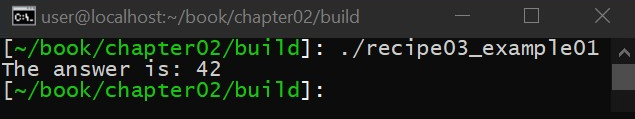In the preceding example, we create a class with both a constructor and a destructor. When we create an instance of the class, the constructor is called, and, when the instance of the class loses scope, the class is destroyed. This is a simple C++ pattern that has been around since the initial versions of C++ were created by Bjarne Stroustrup. Under the hood, the compiler calls a construction function when the class is first instantiated, but, more importantly, the compiler has to inject code into the program that executes the destruction function when the instantiation of the class loses scope. The important thing to understand here is that this additional logic is inserted into the program automatically by the compiler for the programmer.

Before the introduction of the classes, the programmer had to add construction and destruction logic to the program manually, and, while construction is a fairly simple thing to get right, destruction is not. A classic example of this type of issue in C is storing a file handle. The programmer will add a call to an open() function to open the file handle and, when the file is done, will add a call to close() to close the file handle, forgetting to execute the close() function on all possible error cases that might crop up. This is inclusive of when the code is hundreds of lines long and someone new to the program adds another error case, forgetting also to call close() as needed.

RAII solves this issue by ensuring that, once the class loses scope, the resource that was acquired is released, no matter what the control-flow path was. Let's look at the following example:

`#include <iostream>#include <stdexcept>class the_answer{public:    int *answer{};    the_answer() :        answer{new int}    {        *answer = 42;    }    ~the_answer()    {        std::cout << "The answer is: " << *answer << '\n';        delete answer;    }};int main(void){    the_answer is;    if (*is.answer == 42) {        return 0;    }    return 1;}`

In this example, we allocate an integer and initialize it in the constructor of a class. The important thing to notice here is that we do not need to check for nullptr from the new operator. This is because the new operator will throw an exception if the memory allocation fails. If this occurs, not only will the rest of the constructor not be executed, but the object itself will not be constructed. This means if the constructor successfully executed, you know that the instance of the class is in a valid state and actually contains a resource that will be destroyed when the instance of the class loses scope

The destructor of the class then outputs to stdout and deletes the previously allocated memory. The important thing to understand here is that, no matter what control path the code takes, this resource will be released when the instance of the class loses scope. The programmer only needs to worry about the lifetime of the class.

This idea that the lifetime of the resource is directly tied to the lifetime of the object that allocated the resource is important as it solves a complicated issue for the control flow of a program in the presence of C++ exceptions. Let's look at the following example:

`#include <iostream>#include <stdexcept>class the_answer{public:    int *answer{};    the_answer() :        answer{new int}    {        *answer = 43;    }    ~the_answer()    {        std::cout << "The answer is not: " << *answer << '\n';        delete answer;    }};void foo(){    the_answer is;    if (*is.answer == 42) {        return;    }    throw std::runtime_error("");}int main(void){    try {        foo();    }    catch(...)    { }    return 0;}`

In this example, we create the same class as the previous example, but, in our foo() function, we throw an exception. The foo() function, however, doesn't need to catch this exception to ensure that the memory allocated is properly freed. Instead, the destructor handles this for us. In C++, many functions might throw and, without RAII, every single function that could throw would need to be wrapped in a try/catch block to ensure that any resources that were allocated are properly freed. We, in fact, see this pattern a lot in C code, especially in kernel-level programming where goto statements are used to ensure that, within a function, if an error occurs, the function can properly unwind itself to release any resources might have previously been acquired. This result is a nest of code dedicated to checking the result of every function call within the program and the logic needed to properly handle the error.

With this type of programming model, it's no wonder that resource leaks are so common in C. RAII combined with C++ exceptions remove the need for this error-prone logic, resulting in code that is less likely to leak resources.

How RAII is handled in the presence of C++ exceptions is outside the scope of this book as it requires a deeper dive into how C++ exception support is implemented. The important thing to remember is that C++ exceptions are faster than checking the return value of a function for an error (as C++ exceptions are implemented using a no overhead algorithm) but are slow when an actual exception is thrown (as the program has to unwind the stack and properly execute each class destructor as needed). For this reason, and others such as maintainability, C++ exceptions should never be used for valid control flow.

Another way that RAII can be used is the finally pattern, which is provided by the C++ Guideline Support Library (GSL). The finally pattern leverages the destructor-only portion of RAII to provide a simple mechanism to perform non-resource-based cleanup when the control flow of a function is complicated or could throw. Consider the following example:

`#include <iostream>#include <stdexcept>template<typename FUNC>class finally{    FUNC m_func;public:    finally(FUNC func) :        m_func{func}    { }    ~finally()    {        m_func();    }};int main(void){    auto execute_on_exit = finally{[]{        std::cout << "The answer is: 42\n";    }};}`

In the preceding example, we create a class that is capable of storing a lambda function that is executed when an instance of the finally class loses scope. In this particular case, we output to stdout when the finally class is destroyed. Although this uses a pattern similar to that of RAII, this technically is not RAII as no resource has been acquired.

Also, if a resource does need to be acquired, RAII should be used instead of the finally pattern. The finally pattern, instead, is useful when you are not acquiring a resource but want to execute code when a function returns no matter what control flow path the program takes (a conditional branch or C++ exception).

To demonstrate this, let's look at a more complicated example:

`#include <iostream>#include <stdexcept>template<typename FUNC>class finally{    FUNC m_func;public:    finally(FUNC func) :        m_func{func}    { }    ~finally()    {        m_func();    }};int main(void){    try {        auto execute_on_exit = finally{[]{            std::cout << "The answer is: 42\n";        }};        std::cout << "step 1: Collect answers\n";        throw std::runtime_error("???");        std::cout << "step 3: Profit\n";    }    catch (...)    { }}`

When executed, we get the following: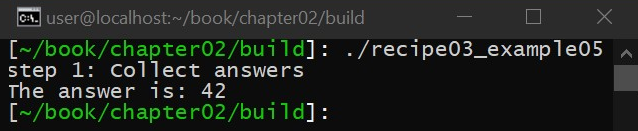In the preceding example, we want to ensure that we always output to stdout no matter what the code does. In the middle of execution, we throw an exception, and even though the exception was thrown, our finally code is executed as intended.

# Learning why to never throw exceptions in destructors

In this recipe, we will discuss the issues with C++ exceptions, specifically in regard to throwing exceptions within a class destructor, something that should be avoided at all costs. The lessons learned in this recipe are important because, unlike other functions, a C++ class destructor is marked as noexcept by default, which means that if you accidentally throw an exception inside a class destructor, your program will call std::terminate(), even though the destructor my not be overtly labeled noexcept.

Before beginning, please ensure that all of the technical requirements are met, including installing Ubuntu 18.04 or higher, and run the following in a Terminal window:

`> sudo apt-get install build-essential git cmake`

This will ensure your operating system has the proper tools to compile and execute the examples in this recipe. Once this is complete, open a new Terminal. We will use this Terminal to download, compile, and run our examples.

# How to do it...

Perform the following steps to try the recipe:

1. From a new Terminal, run the following to download the source code:
`> cd ~/> git clone https://github.com/PacktPublishing/Advanced-CPP-CookBook.git> cd Advanced-CPP-CookBook/chapter02`
1. To compile the source code, run the following:
`> mkdir build && cd build> cmake ..> make recipe04_examples`
1. Once the source code is compiled, you can execute each example in this recipe by running the following commands:
`> ./recipe04_example01terminate called after throwing an instance of 'std::runtime_error'what(): 42Aborted> ./recipe04_example02The answer is: 42> ./recipe04_example03terminate called after throwing an instance of 'std::runtime_error'what(): 42Aborted> ./recipe04_example04# exceptions: 2The answer is: 42The answer is: always 42`

In the next section, we will step through each of these examples and explain what each example program does and how it relates to the lessons being taught in this recipe.

# How it works...

In this recipe, we will learn why throwing exceptions in a destructor is a bad idea, and why class destructors are labeled as noexcept by default. To start, let's look at a simple example:

`#include <iostream>#include <stdexcept>class the_answer{public:    ~the_answer()    {        throw std::runtime_error("42");    }};int main(void){    try {        the_answer is;    }    catch (const std::exception &e) {        std::cout << "The answer is: " << e.what() << '\n';    }}`

When we execute this, we get the following: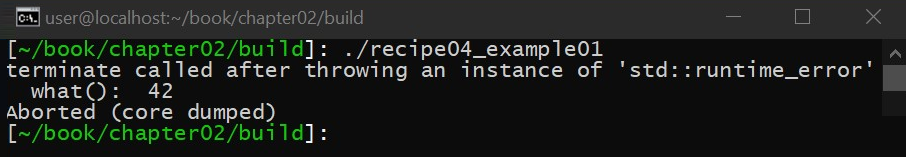In this example, we can see that if we throw an exception from a class destructor, std::terminate() is called. This is because, by default, a class destructor is marked as noexcept.

We can change this by explicitly allowing a class destructor to throw by marking the class's destructor as noexcept(false), as shown in the next example:

`#include <iostream>#include <stdexcept>class the_answer{public:    ~the_answer() noexcept(false)    {        throw std::runtime_error("42");    }};int main(void){    try {        the_answer is; }    catch (const std::exception &e) {        std::cout << "The answer is: " << e.what() << '\n';    }}`

As shown in the preceding example, when the class is destroyed, an exception is thrown and properly handled. Even though this was successfully handled, we have to ask ourselves, what is the state of the program after we catch this exception? The destructor didn't successfully complete. If this class was more complex and had state/resources that it was managing, can we conclude that the state/resources that we care about were properly handled/released? The short answer is no. This is the same as destroying a hard drive with a hammer. If you slam a hard drive with a hammer to destroy it, did you actually destroy the data on the hard drive? There is no way to know because, when you hit the hard drive with the hammer, you broke the electronics that would have been used to answer that very question. When you attempt to destroy a hard drive, you need a reliable process that ensures that, under no circumstance could the process of destroying the drive leave data in a recoverable state. Otherwise, you have no way of knowing what state you are in, with no way of going back.

The same applies to C++ classes. Destroying a C++ class needs to be an operation that must provide basic exception safety (that is, the state of the program is deterministic with some possible side effects). Otherwise, the only other logical course of action is to call std::terminate() since you cannot be sure what will happen if the program continues to execute.

Besides putting the program in an undefined state, the other issue with throwing an exception from a destructor is, what happens if an exception has already been thrown? What does the try/catch block catch? Let's look at an example of this type of issue:

`#include <iostream>#include <stdexcept>class the_answer{public:    ~the_answer() noexcept(false)    {        throw std::runtime_error("42");    }};int main(void){    try {        the_answer is;        throw std::runtime_error("first exception");    }    catch (const std::exception &e) {        std::cout << "The answer is: " << e.what() << '\n';    }}`

In the preceding example, we mark the destructor as noexcept(false) just like we did in the previous example, but we throw before the destructor is called, which means that, when the destructor is called, there is already an exception being processed. Now, when we attempt to throw, std::terminate() is called even though the destructor was marked as noexcept(false):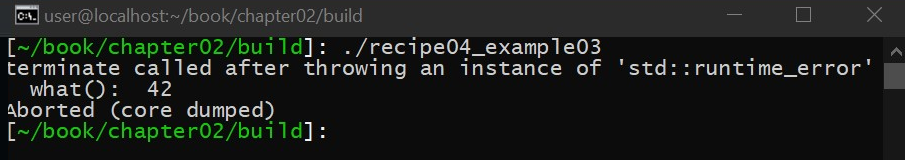The reason for this is the C++ library has no way of handling this situation because the try/catch block cannot handle more than one exception. It is possible, however, to have more than one pending exception; we simply need a try/catch block to handle each exception. This situation occurs when we have nested exceptions, as in this example:

`#include <iostream>#include <stdexcept>class nested{public:    ~nested()    {        std::cout << "# exceptions: " << std::uncaught_exceptions() << '\n';    }};class the_answer{public:    ~the_answer()    {        try {            nested n;            throw std::runtime_error("42");        }        catch (const std::exception &e) {            std::cout << "The answer is: " << e.what() << '\n';        }    }};`

In this example, we will start by creating a class that outputs the results of calling std::uncaught_exceptions(), which returns the total number of exceptions currently being processed. We will then create a second class that creates the first class and then throws from its destructor, with the important note that all of the code in the destructor is wrapped in a try/catch block:

`int main(void){    try {        the_answer is;        throw std::runtime_error("always 42");    }    catch (const std::exception &e) {        std::cout << "The answer is: " << e.what() << '\n';    }}`

When this example is executed, we get the following: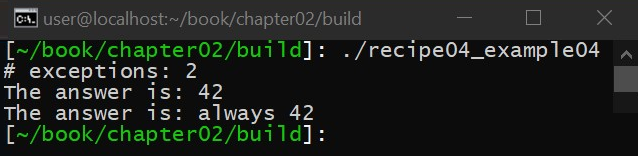Finally, we will create this second class and throw again with another try/catch block. Unlike the previous example, all of the exceptions are being properly handled, and, in fact, noexcept(false) is not needed to ensure that this code executes properly as, for each exception that is thrown, we have a try/catch block. Even though an exception was thrown inside a destructor, it was properly handled, which means that the destructor executes safely and remains noexcept-compliant, even though the second class is executing in the presence of the two exceptions being processed.

# Easily creating your own exception classes

In this recipe, you will learn how to easily create your own exception types. This is an important lesson to learn as, although C++ exceptions are easy to create yourself, some guidelines should be followed to ensure this is done safely.

Before beginning, please ensure that all of the technical requirements are met, including installing Ubuntu 18.04 or higher and running the following in a Terminal window:

`> sudo apt-get install build-essential git cmake`

This will ensure your operating system has the proper tools to compile and execute the examples in this recipe. Once this is complete, open a new Terminal. We will use this Terminal to download, compile, and run our examples.

# How to do it...

Perform the following steps to try the recipe:

1. From a new Terminal, run the following to download the source code:
`> cd ~/> git clone https://github.com/PacktPublishing/Advanced-CPP-CookBook.git> cd Advanced-CPP-CookBook/chapter02`
1. To compile the source code, run the following:
`> mkdir build && cd build> cmake ..> make recipe05_examples`
1. Once the source code is compiled, you can execute each example in this recipe by running the following commands:
`> ./recipe05_example01The answer is: 42> ./recipe05_example02The answer is: 42> ./recipe05_example03The answer is: 42`

In the next section, we will step through each of these examples and explain what each example program does and how it relates to the lessons being taught in this recipe.

# How it works...

Creating your own C++ exceptions allows you to filter out what type of exception you are getting. For example, did the exception come from your code or the C++ library? By creating your own C++ exceptions, you can easily answer these questions during runtime in your own code. Let's look at the following example:

`#include <iostream>#include <stdexcept>class the_answer : public std::exception{public:    the_answer() = default;    const char *what() const noexcept    {        return "The answer is: 42";    }};int main(void){    try {        throw the_answer{};    }    catch (const std::exception &e) {        std::cout << e.what() << '\n';    }}`

As shown in the preceding example, we create our own C++ exception by inheriting std::exception. This is not a requirement. Technically, anything can be a C++ exception including an integer. Starting from std::exception, however, gives you a standard interface to work from including overriding the what() function, which describes the exception that was thrown.

In this preceding example, we return a hardcoded string in the what() function. This is the ideal type of exception (even more so than the exceptions that are provided by the C++ library). This is because this type of exception is nothrow copy-constructable. Specifically, this means that the exception itself can be copied without the copy generating an exception, for example, due to std::bad_alloc. The exception types provided by the C++ library support construction from std::string(), which could throw std::bad_alloc.

The issue with the preceding C++ exception is that you would need 1 exception type for every type of message you wish to provide. Another way to implement a safe exception type is to use the following:

`#include <iostream>#include <stdexcept>class the_answer : public std::exception{    const char *m_str;public:    the_answer(const char *str):        m_str{str}    { }    const char *what() const noexcept    {        return m_str;    }};int main(void){    try {        throw the_answer("42");    }    catch (const std::exception &e) {        std::cout << "The answer is: " << e.what() << '\n';    }}`

In the preceding example, we store a pointer to const char* (that is, a C-style string). C-style strings are stored globally as constants within the program. This type of exception satisfies all of the same preceding rules, and no allocations are taking place during the construction of the exception. It should also be noted that, since the strings are stored globally, this type of operation is safe.

Many types of exceptions can be created using this approach, including things other than strings that are accessible through custom getters (that is, without having to use the what() function). If, however, these preceding rules are not an issue for you, the easiest way to create a custom C++ exception is to simply subclass an existing C++ exception such as std::runtime_error(), as in the following example:

`#include <iostream>#include <stdexcept>#include <string.h>class the_answer : public std::runtime_error{public:    explicit the_answer(const char *str) :        std::runtime_error{str}    { }};int main(void){    try {        throw the_answer("42");    }    catch (const the_answer &e) {        std::cout << "The answer is: " << e.what() << '\n';    }    catch (const std::exception &e) {        std::cout << "unknown exception: " << e.what() << '\n';    }}`

When this example is executed, we get the following: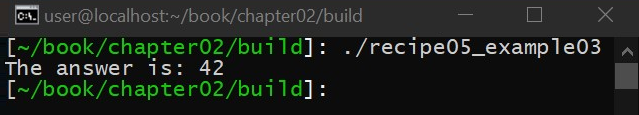In the preceding example, we create our own C++ exception in just a few lines of code by subclassing std::runtime_error(). We can then use different catch blocks to figure out what type of exception was thrown. Just remember that if you use the std::string version of std::runtime_error(), you could end up with std::bad_alloc being thrown during the construction of the exception itself.